Module 14 - Related Rates

Introduction | Lesson 1 | Lesson 2 | Lesson 3 | Lesson 4 | Self-Test

Lesson 14.3: Two Ships

A related-rate problem that models two ships as they move away from each other is discussed in this lesson.

Two ships start at a point O and move away from that point along routes that make a 120° angle. Ship A moves at 14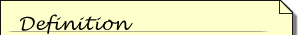A knot is a unit used to measure the speed of a ship. One knot represents one nautical mile (6,076.1 feet) an hour.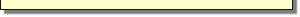knots and Ship B moves at 21 knots. In the diagram below A represents the position of Ship A, B represents the position of Ship B, and c represents the distance between the ships.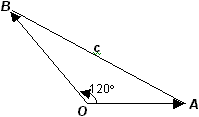Modeling the Positions of the Ships

Suppose that Ship A is moving along the positive x-axis at 14 knots. Enter the parametric equations for Ship A's position at time t hours.

• Set xt1 = 14t
• Set yt1 = 0

Parametric equations can be used to model the position of an object at time t that is moving at speed v along a line that forms an angle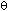with the positive x-axis. Such equations have the form

x = vt cosy = vt sinEnter the equations for Ship B's position, which is moving at 21 knots along a line that forms a 120° angle with the path of Ship A.

• Set Angle mode to DEGREE
• Set xt2 = 21t*cos(120)
• Set yt2 = 21t*sin(120)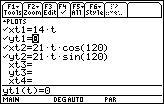Animating the Motion of the Ships

• Change the Graph Style of both sets of equations to "Path"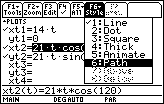Make sure the Graph Order in the Graph Formats dialog box is still Simultaneous.

• Enter the Viewing Window values shown below: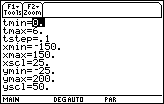• Graph the equations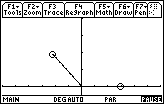Finding the Distance between the Ships

The distance c(t) between the ships can be found by using the distance formula: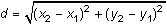Use the restriction t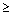0 because the problem starts at t = 0.

• Define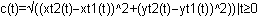• Enter c(t) on the Edit Line and press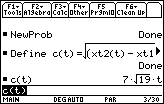Thus,.

Finding the Speed at which the Ships are Moving Apart

The speed at which the ships are moving apart can be found by finding the derivative of c(t) with respect to t, or just observing that it is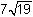knots from the formula for c(t).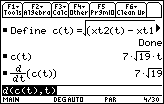Modifying the Problem

Assume that both ships travel in the same direction and at the same speed as before, but Ship A begins its journey 5 nautical miles from point O and Ship B begins 3 nautical miles from point O.

• Set    xt1 with 14t+5
xt2 with (21t+3)*cos(120)
yt2 with (21t+3)*sin(120)